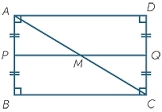# Perimeter 11631

The length of the garden measures 27 1/4m. How many meters is this dimension larger than the width of the garden, which measures 15 1/2 m? Calculate the perimeter of the garden.

d =  11 34 = 47/4 m
o =  85 12 = 171/2 m

### Step-by-step explanation:Did you find an error or inaccuracy? Feel free to write us. Thank you!

Tips for related online calculators
Need help calculating sum, simplifying, or multiplying fractions? Try our fraction calculator.
Need help with mixed numbers? Try our mixed-number calculator.

#### Grade of the word problem:

We encourage you to watch this tutorial video on this math problem: